# Example Deep Learning Networks Architectures

This example shows how to define simple deep learning neural networks for classification and regression tasks.

The networks in this example are basic networks that you can modify for your task. For example, some networks have sections that you can replace with deeper sections of layers that can better learn from and process the data for your task.

The descriptions of the networks specify the format of the data that flows through the network using a string of characters representing the different dimensions of the data. The formats contain one or more of these characters:

• `"S"` — Spatial

• `"C"` — Channel

• `"B"` — Batch

• `"T"` — Time

• `"U"` — Unspecified

For example, you can represent 2-D image data as a 4-D array, in which the first two dimensions correspond to the spatial dimensions of the images, the third dimension corresponds to the channels of the images, and the fourth dimension corresponds to the batch dimension. This representation is in the format `"SSCB"` (spatial, spatial, channel, batch).

### Image Data

Image data typically has two or three spatial dimensions.

• 2-D image data is typically represented in the format `"SSCB"` (spatial, spatial, channel, batch).

• 3-D image data is typically represented in the format `"SSSCB"` (spatial, spatial, spatial, channel, batch).

#### 2-D Image Classification Network

A 2-D image classification network maps `"SSCB"` (spatial, spatial, channel, batch) data to `"CB"` (channel, batch) data and then passes the mapped data to a classification layer.

The fully connected layer processes the data so that the `"C"` (channel) dimension of the network output matches the number of classes. The softmax layer converts its input data to vectors of probabilities for classification.

```inputSize = [224 224 3]; numClasses = 10; filterSize = 3; numFilters = 128; layers = [ imageInputLayer(inputSize) convolution2dLayer(filterSize,numFilters) batchNormalizationLayer reluLayer fullyConnectedLayer(numClasses) softmaxLayer classificationLayer];```

You can replace the convolution, batch normalization, ReLU layer block with a block of layers that processes 2-D image data. This block maps `"SSCB"` (spatial, spatial, channel, batch) data to `"SSCB"` (spatial, spatial, channel, batch) data.

For an example that shows how to train a neural network for image classification, see Create Simple Deep Learning Neural Network for Classification.

#### 2-D Image Regression Network

A 2-D image regression network maps `"SSCB"` (spatial, spatial, channel, batch) data to `"CB"` (channel, batch) data and then passes the mapped data to a regression layer.

The fully connected layer processes the data so that the `"C"` (channel) dimension of the network output matches the number of responses.

```inputSize = [224 224 3]; numResponses = 10; filterSize = 3; numFilters = 128; layers = [ imageInputLayer(inputSize) convolution2dLayer(filterSize,numFilters) batchNormalizationLayer reluLayer fullyConnectedLayer(numResponses) regressionLayer];```

You can replace the convolution, batch normalization, ReLU layer block with a block of layers that processes 2-D image data. This block maps `"SSCB"` (spatial, spatial, channel, batch) data to `"SSCB"` (spatial, spatial, channel, batch) data.

For an example that shows how to train a neural network for image regression, see Train Convolutional Neural Network for Regression.

#### 2-D Image-to-Image Regression Network

A 2-D image-to-image regression network maps `"SSCB"` (spatial, spatial, channel, batch) data to `"SSCB"` (spatial, spatial, channel, batch) data and then passes the mapped data to a regression layer.

The network downsamples the data using a max pooling layer with a stride of two. The network upsamples the downsampled data using a transposed convolution layer.

The final convolution layer processes the data so that the `"C"` (channel) dimension of the network output matches the number of output channels. The clipped ReLU layer clips its input so that the network outputs data in the range [0, 1].

```inputSize = [224 224 3]; numOutputChannels = 3; filterSize = 3; numFilters = 128; layers = [ imageInputLayer(inputSize) convolution2dLayer(filterSize,numFilters,Padding="same") reluLayer maxPooling2dLayer(2,Padding="same",Stride=2) transposedConv2dLayer(filterSize,numFilters,Stride=2) reluLayer convolution2dLayer(1,numOutputChannels,Padding="same") clippedReluLayer(1) regressionLayer];```

You can replace the convolution, ReLU, max pooling layer block with a block of layers that downsamples 2-D image data. This block maps `"SSCB"` (spatial, spatial, channel, batch) data to `"SSCB"` (spatial, spatial, channel, batch) data.

You can replace the transposed convolution, ReLU layer block with a block of layers that upsamples 2-D image data. This block maps `"SSCB"` (spatial, spatial, channel, batch) data to `"SSCB"` (spatial, spatial, channel, batch) data.

For an example that shows how to train a neural network for image-to-image regression, see Prepare Datastore for Image-to-Image Regression.

#### 3-D Image Classification Network

A 3-D image classification network maps `"SSSCB"` (spatial, spatial, spatial, channel, batch) data to `"CB"` (channel, batch) data and then passes the mapped data to a classification layer.

The fully connected layer processes the data so that the `"C"` (channel) dimension of the network output matches the number of classes. The softmax layer converts its input data to vectors of probabilities for classification.

```inputSize = [224 224 224 3]; numClasses = 10; filterSize = 3; numFilters = 128; layers = [ image3dInputLayer(inputSize) convolution3dLayer(filterSize,numFilters) batchNormalizationLayer reluLayer fullyConnectedLayer(numClasses) softmaxLayer classificationLayer];```

You can replace the convolution, batch normalization, ReLU layer block with a block of layers that processes 3-D image data. This block maps `"SSSCB"` (spatial, spatial, spatial, channel, batch) data to `"SSSCB"` (spatial, spatial, spatial, channel, batch) data.

#### 3-D Image Regression Network

A 3-D image regression network maps `"SSSCB"` (spatial, spatial, spatial, channel, batch) data to `"CB"` (channel, batch) data and then passes the mapped data to a regression layer.

The fully connected layer processes the data so that the `"C"` (channel) dimension of the network output matches the number of responses.

```inputSize = [224 224 224 3]; numResponses = 10; filterSize = 3; numFilters = 128; layers = [ image3dInputLayer(inputSize) convolution3dLayer(filterSize,numFilters) batchNormalizationLayer reluLayer fullyConnectedLayer(numResponses) regressionLayer];```

You can replace the convolution, batch normalization, ReLU layer block with a block of layers that processes 3-D image data. This block maps `"SSSCB"` (spatial, spatial, spatial, channel, batch) data to `"SSSCB"` (spatial, spatial, spatial, channel, batch) data.

### Sequence Data

Sequence data typically has a time dimension.

• Vector sequence data is typically represented in the format `"CBT"` (channel, batch, time).

• 2-D image sequence data is typically represented in the format `"SSCBT"` (spatial, spatial, channel, batch, time).

• 3-D image sequence data is typically represented in the format `"SSSCBT"` (spatial, spatial, spatial, channel, batch, time).

#### Vector Sequence-to-Label Classification Network

A vector sequence-to-label classification network maps `"CBT"` (channel, batch, time) data to `"CB"` (channel, batch) data and then passes the mapped data to a classification layer.

#### LSTM Network

When the `OutputMode` option of the LSTM layer is `"last"`, the layer outputs only the last time step of the data in the format `"CB"` (channel, batch).

The fully connected layer processes the data so that the `"C"` (channel) dimension of the network output matches the number of classes. The softmax layer converts its input data to vectors of probabilities for classification.

```numFeatures = 15; numClasses = 10; numHiddenUnits = 100; layers = [ sequenceInputLayer(numFeatures) lstmLayer(numHiddenUnits,OutputMode="last") fullyConnectedLayer(numClasses) softmaxLayer classificationLayer];```

You can replace the LSTM layer with a block of layers that process vector sequence data. This layer maps `"CBT"` (channel, batch, time) data to `"CB"` (channel, batch) data.

For an example that shows how to train an LSTM network for classification, see Sequence Classification Using Deep Learning.

#### Convolutional Network

The 1-D convolution layer convolves over the `"T"` (time) dimension of its input data. The 1-D global max pooling layer maps `"CBT"` (channel, batch, time) data to `"CB"` (channel, batch) data.

The fully connected layer processes the data so that the `"C"` (channel) dimension of the network output matches the number of classes. The softmax layer converts its input data to vectors of probabilities for classification.

```numFeatures = 15; numClasses = 10; minLength = 100; filterSize = 3; numFilters = 128; layers = [ sequenceInputLayer(numFeatures,MinLength=minLength) convolution1dLayer(filterSize,numFilters) batchNormalizationLayer reluLayer globalMaxPooling1dLayer fullyConnectedLayer(numClasses) softmaxLayer classificationLayer];```

You can replace the convolution, batch normalization, ReLU layer block with a block of layers that processes sequence data. This block maps `"CBT"` (channel, batch, time) data to `"CBT"` (channel, batch, time) data.

For an example that shows how to train a classification network using 1-D convolutions, see Sequence Classification Using 1-D Convolutions.

#### Vector Sequence-to-One Regression Network

A vector sequence-to-one regression network maps `"CBT"` (channel, batch, time) data to `"CB"` (channel, batch) data and then passes the mapped data to a regression layer.

When the `OutputMode` option of the LSTM layer is `"last"`, the layer outputs only the last time step of the data in the format `"CB"` (channel, batch).

The fully connected layer processes the data so that the `"C"` (channel) dimension of the network output matches the number of responses.

```numFeatures = 15; numResponses = 10; numHiddenUnits = 100; layers = [ sequenceInputLayer(numFeatures) lstmLayer(numHiddenUnits,OutputMode="last") fullyConnectedLayer(numResponses) regressionLayer];```

You can replace the LSTM layer with a block of layers that processes vector sequence data. This layer maps `"CBT"` (channel, batch, time) data to `"CB"` (channel, batch) data.

For an example that shows how to train an LSTM network for regression, see Sequence-to-One Regression Using Deep Learning.

#### Vector Sequence-to-Sequence Classification Network

A vector sequence-to-sequence classification network maps `"CBT"` (channel, batch, time) data to `"CBT"` (channel, batch, time) data and then passes the mapped data to a classification layer.

When the `OutputMode` option of the LSTM layer is `"sequence"`, the layer outputs all the time steps of the data in the format `"CBT"` (channel, batch, time).

The fully connected layer processes the data so that the `"C"` (channel) dimension of the network output matches the number of classes. The softmax layer converts the time steps of its input data to vectors of probabilities for classification.

```numFeatures = 15; numClasses = 10; numHiddenUnits = 100; layers = [ sequenceInputLayer(numFeatures) lstmLayer(numHiddenUnits) fullyConnectedLayer(numClasses) softmaxLayer classificationLayer];```

You can replace the LSTM layer with a block of layers that processes vector sequence data. This layer maps `"CBT"` (channel, batch, time) data to `"CBT"` (channel, batch, time) data.

For an example that shows how to train an LSTM network for sequence-to-sequence classification, see Sequence-to-Sequence Classification Using Deep Learning.

#### Vector Sequence-to-Sequence Regression Network

A vector sequence-to-sequence regression network maps `"CBT"` (channel, batch, time) data to `"CBT"` (channel, batch, time) data and then passes the mapped data to a regression layer.

When the `OutputMode` option of the LSTM layer is `"sequence"`, the layer outputs all the time steps of the data in the format `"CBT"` (channel, batch, time).

The fully connected layer processes the data so that the `"C"` (channel) dimension of the network output matches the number of responses.

```numFeatures = 15; numResponses = 10; numHiddenUnits = 100; layers = [ sequenceInputLayer(numFeatures) lstmLayer(numHiddenUnits) fullyConnectedLayer(numResponses) regressionLayer];```

You can replace the LSTM layer with a block of layers that processes vector sequence data. This layer maps `"CBT"` (channel, batch, time) data to `"CBT"` (channel, batch, time) data.

For an example that shows how to train a sequence-to-sequence regression network, see Sequence-to-Sequence Regression Using Deep Learning.

#### Image Sequence-to-Label Classification Network

An image sequence-to-label classification network maps `"SSCBT"` (spatial, spatial, channel, batch, time) data to `"CB"` data (channel, batch) and then passes the mapped data to a classification layer.

The convolution layer processes the frames independently. To map the processed frames to vector sequence data, the network uses a flatten layer that maps `"SSCBT"` (spatial, spatial, channel, batch, time) data to `"CBT"` (channel, batch, time) data.

When the `OutputMode` option of the LSTM layer is `"last"`, the layer outputs only the last time step of the data in the format `"CB"` (channel, batch).

The fully connected layer processes the data so that the `"C"` (channel) dimension of the network output matches the number of classes. The softmax layer converts the time steps of its input data to vectors of probabilities for classification.

```inputSize = [224 224 3]; numClasses = 10; numHiddenUnits = 100; filterSize = 3; numFilters = 224; layers = [ sequenceInputLayer(inputSize) convolution2dLayer(filterSize,numFilters) batchNormalizationLayer reluLayer flattenLayer lstmLayer(numHiddenUnits,OutputMode="last") fullyConnectedLayer(numClasses) softmaxLayer classificationLayer];```

You can replace the convolution, batch normalization, ReLU layer block with a block of layers that processes sequences of 2-D images. This block maps `"SSCBT"` (spatial, spatial, channel, batch, time) data to `"SSCBT"` (spatial, spatial, channel, batch, time) data.

You can replace the LSTM layer with a block of layers that processes vector sequence data. This layer maps `"CBT"` (channel, batch, time) data to `"CB"` (channel, batch) data.

For image sequence-to-sequence classification, for example, per-frame video classification, set the `OutputMode` option of the LSTM layer to `"sequence"`.

For an example that shows how to train an image sequence-to-label classification network for video classification, see Classify Videos Using Deep Learning.

#### Image Sequence-to-One Regression Network

An image sequence-to-one regression network maps `"SSCBT"` (spatial, spatial, channel, batch, time) data to `"CB"` data (channel, batch) and then passes the mapped data to a regression layer.

The convolution layer processes the frames independently. To map the processed frames to vector sequence data, the network uses a flatten layer that maps `"SSCBT"` (spatial, spatial, channel, batch, time) data to `"CBT"` (channel, batch, time) data.

When the `OutputMode` option of the LSTM layer is `"last"`, the layer outputs only the last time step of the data in the format `"CB"` (channel, batch).

The fully connected layer processes the data so that the `"C"` (channel) dimension of the network output matches the number of responses.

```inputSize = [224 224 3]; numResponses = 10; numHiddenUnits = 100; filterSize = 3; numFilters = 224; layers = [ sequenceInputLayer(inputSize) convolution2dLayer(filterSize,numFilters) batchNormalizationLayer reluLayer flattenLayer lstmLayer(numHiddenUnits,OutputMode="last") fullyConnectedLayer(numResponses) regressionLayer];```

You can replace the convolution, batch normalization, ReLU layer block with a block of layers that processes sequences of 2-D images. This block maps `"SSCBT"` (spatial, spatial, channel, batch, time) data to `"SSCBT"` (spatial, spatial, channel, batch, time) data.

You can replace the LSTM layer with a block of layers that processes vector sequence data. This layer maps `"CBT"` (channel, batch, time) data to `"CB"` (channel, batch) data.

For image sequence-to-sequence regression, for example, per-frame video regression, set the `OutputMode` option of the LSTM layer to `"sequence"`.

### Feature Data

Feature data is typically represented in the format `"CB"` (channel, batch).

#### Feature Classification Network

A feature classification network maps `"CB"` (channel, batch) data to `"CB"` (channel, batch) data and then passes the mapped data to a classification layer.

#### Multilayer Perceptron Classification Network

The fully connected layer processes the data so that the `"C"` (channel) dimension of the network output matches the number of classes. The softmax layer converts its input data to vectors of probabilities for classification.

```numFeatures = 15; numClasses = 10; hiddenSize = 100; layers = [ featureInputLayer(numFeatures) fullyConnectedLayer(hiddenSize) reluLayer fullyConnectedLayer(numClasses) softmaxLayer classificationLayer];```

You can replace the first fully connected layer and ReLU layer with a block of layers that processes feature data. This block maps `"CB"` (channel, batch) data to `"CB"` (channel, batch) data.

For an example that shows how to train a feature classification network, see Train Network with Numeric Features.

#### Feature Regression Network

A feature regression network maps `"CB"` (channel, batch) data to `"CB"` data (channel, batch) and then passes the mapped data to a regression layer.

#### Multilayer Perceptron Regression Network

The fully connected layer processes the data so that the `"C"` (channel) dimension of the network output matches the number of responses.

```numFeatures = 15; numResponses = 10; hiddenSize = 100; layers = [ featureInputLayer(numFeatures) fullyConnectedLayer(hiddenSize) reluLayer fullyConnectedLayer(numResponses) regressionLayer];```

You can replace the first fully connected layer and ReLU layer with a block of layers that processes feature data. This block maps `"CB"` (channel, batch) data to `"CB"` (channel, batch) data.

### Multiple Input Networks

Neural networks can have multiple inputs. Networks with multiple inputs typically process data from different sources and merge the processed data using a combination layer such as an addition layer or a concatenation layer.

#### Multiple 2-D Image Input Classification Network

A multiple 2-D image input classification network maps `"SSCB"` (spatial, spatial, channel, batch) data from multiple sources to `"CB"` (channel, batch) data and then passes the mapped data to a classification layer.

The flatten layers map `"SSCB"` (spatial, spatial, channel, batch) data to `"CB"` (channel, batch) data. The concatenation layer concatenates two inputs in the format `"CB"` (channel, batch) along the `"C"` (channel) dimension. The fully connected layer processes the data so that the `"C"` (channel) dimension of the network output matches the number of classes. The softmax layer converts its input data to vectors of probabilities for classification.

```inputSize1 = [224 224 3]; inputSize2 = [64 64 1]; numClasses = 10; filterSize1 = 5; numFilters1 = 128; filterSize2 = 3; numFilters2 = 64; layers = [ imageInputLayer(inputSize1) convolution2dLayer(filterSize1,numFilters1) batchNormalizationLayer reluLayer flattenLayer concatenationLayer(1,2,Name="cat") fullyConnectedLayer(numClasses) softmaxLayer classificationLayer]; lgraph = layerGraph(layers); layers = [ imageInputLayer(inputSize2) convolution2dLayer(filterSize2,numFilters2) batchNormalizationLayer reluLayer flattenLayer(Name="flatten2")]; lgraph = addLayers(lgraph,layers); lgraph = connectLayers(lgraph,"flatten2","cat/in2"); figure plot(lgraph)```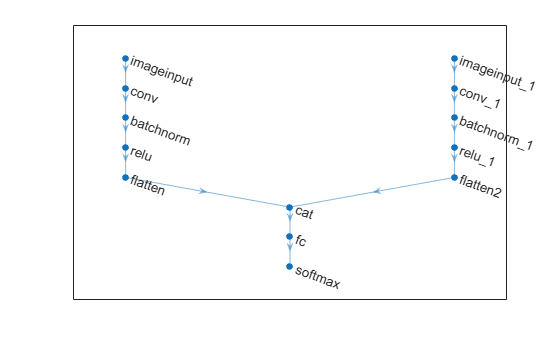You can replace the convolution, batch normalization, ReLU layer blocks with blocks of layers that process 2-D image data. These blocks map `"SSCB"` (spatial, spatial, channel, batch) data to `"SSCB"` (spatial, spatial, channel, batch) data.

#### Multiple 2-D Image Input Regression Network

A multiple 2-D image input regression network maps `"SSCB"` (spatial, spatial, channel, batch) data from multiple sources to `"CB"` (channel, batch) data and then passes the mapped data to a regression layer.

The flatten layers map `"SSCB"` (spatial, spatial, channel, batch) data to `"CB"` (channel, batch) data. The concatenation layer concatenates two inputs in the format `"CB"` (channel, batch) along the `"C"` (channel) dimension. The fully connected layer processes the data so that the `"C"` (channel) dimension of the network output matches the number of responses.

```inputSize1 = [224 224 3]; inputSize2 = [64 64 1]; numResponses = 10; filterSize1 = 5; numFilters1 = 128; filterSize2 = 3; numFilters2 = 64; layers = [ imageInputLayer(inputSize1) convolution2dLayer(filterSize1,numFilters1) batchNormalizationLayer reluLayer flattenLayer concatenationLayer(1,2,Name="cat") fullyConnectedLayer(numResponses) regressionLayer]; lgraph = layerGraph(layers); layers = [ imageInputLayer(inputSize2) convolution2dLayer(filterSize2,numFilters2) batchNormalizationLayer reluLayer flattenLayer(Name="flatten2")]; lgraph = addLayers(lgraph,layers); lgraph = connectLayers(lgraph,"flatten2","cat/in2"); figure plot(lgraph)```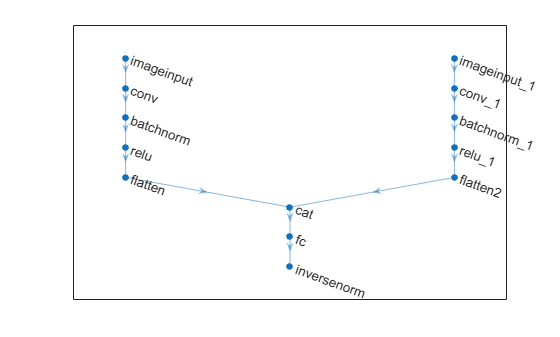You can replace the convolution, batch normalization, ReLU layer blocks with blocks of layers that process 2-D image data. These blocks map `"SSCB"` (spatial, spatial, channel, batch) data to `"SSCB"` (spatial, spatial, channel, batch) data.

#### 2-D Image and Feature Classification Network

A 2-D image and feature classification network maps one input of `"SSCB"` (spatial, spatial, channel, batch) data and one input of `"CB"` (channel, batch) data to `"CB"` (channel, batch) data and then passes the mapped data to a classification layer.

The flatten layer maps `"SSCB"` (spatial, spatial, channel, batch) data to `"CB"` (channel, batch) data. The concatenation layer concatenates two inputs in the format `"CB"` (channel, batch) along the `"C"` (channel) dimension. The fully connected layer processes the data so that the `"C"` (channel) dimension of the network output matches the number of classes. The softmax layer converts its input data to vectors of probabilities for classification.

```inputSize = [224 224 3]; numFeatures = 15; numClasses = 10; filterSize = 5; numFilters = 128; hiddenSize = 100; layers = [ imageInputLayer(inputSize) convolution2dLayer(filterSize,numFilters) batchNormalizationLayer reluLayer flattenLayer concatenationLayer(1,2,Name="cat") fullyConnectedLayer(numClasses) softmaxLayer classificationLayer]; lgraph = layerGraph(layers); layers = [ featureInputLayer(numFeatures) fullyConnectedLayer(hiddenSize) reluLayer(Name="relu2")]; lgraph = addLayers(lgraph,layers); lgraph = connectLayers(lgraph,"relu2","cat/in2"); figure plot(lgraph)```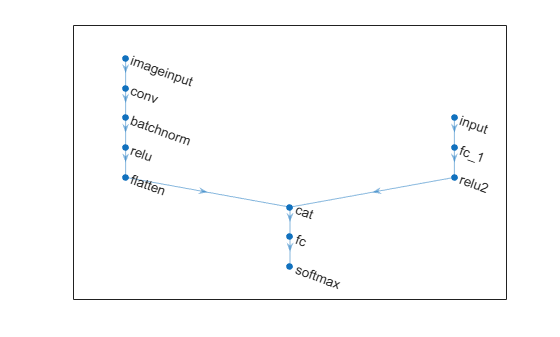You can replace the convolution, batch normalization, ReLU layer block with a block of layers that processes 2-D image data. This block maps `"SSCB"` (spatial, spatial, channel, batch) data to `"SSCB"` (spatial, spatial, channel, batch) data.

You can replace the fully connected layer and ReLU layer in the feature branch with a block of layers that processes feature data. This block maps `"CB"` (channel, batch) data to `"CB"` (channel, batch) data.

For an example that shows how to train a network on image and feature data, see Train Network on Image and Feature Data.

#### 2-D Image and Vector-Sequence Classification Network

A 2-D image and vector-sequence classification network maps one input of `"SSCB"` (spatial, spatial, channel, batch) data and one input of `"CBT"` (channel, batch, time) data to `"CB"` (channel, batch) data and then passes the mapped data to a classification layer.

The flatten layer maps `"SSCB"` (spatial, spatial, channel, batch) data to `"CB"` (channel, batch) data. When the `OutputMode` option of the LSTM layer is `"last"`, the layer outputs only the last time step of the data in the format `"CB"` (channel, batch). The concatenation layer concatenates two inputs in the format `"CB"` (channel, batch) along the `"C"` (channel) dimension. The fully connected layer processes the data so that the `"C"` (channel) dimension of the network output matches the number of classes. The softmax layer converts its input data to vectors of probabilities for classification.

```inputSize = [224 224 3]; numFeatures = 15; numClasses = 10; filterSize = 5; numFilters = 128; numHiddenUnits = 100; layers = [ imageInputLayer(inputSize) convolution2dLayer(filterSize,numFilters) batchNormalizationLayer reluLayer flattenLayer concatenationLayer(1,2,Name="cat") fullyConnectedLayer(numClasses) softmaxLayer classificationLayer]; lgraph = layerGraph(layers); layers = [ sequenceInputLayer(numFeatures) lstmLayer(hiddenSize,OutputMode="last",Name="lstm")]; lgraph = addLayers(lgraph,layers); lgraph = connectLayers(lgraph,"lstm","cat/in2"); figure plot(lgraph)```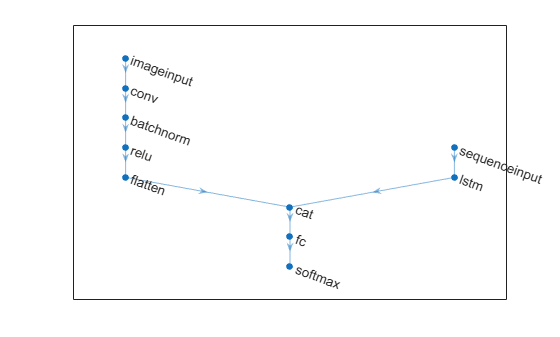You can replace the convolution, batch normalization, ReLU layer block with a block of layers that processes 2-D image data. This block maps `"SSCB"` (spatial, spatial, channel, batch) data to `"SSCB"` (spatial, spatial, channel, batch) data.

You can replace the LSTM layer with a block of layers that processes vector sequence data. This layer maps `"CBT"` (channel, batch, time) data to `"CB"` (channel, batch) data.

#### Vector-Sequence and Feature Classification Network

A vector-sequence and feature classification network maps one input of `"CBT"` (channel, batch, time) data and one input of `"CB"` (channel, batch) data to `"CB"` (channel, batch) data and then passes the mapped data to a classification layer.

When the `OutputMode` option of the LSTM layer is `"last"`, the layer outputs only the last time step of the data in the format `"CB"` (channel, batch). The concatenation layer concatenates two inputs in the format `"CB"` (channel, batch) along the `"C"` (channel) dimension. The fully connected layer processes the data so that the `"C"` (channel) dimension of the network output matches the number of classes. The softmax layer converts its input data to vectors of probabilities for classification.

```numFeatures = 15; numFeaturesSequence = 20; numClasses = 10; numHiddenUnits = 128; hiddenSize = 100; layers = [ sequenceInputLayer(numFeaturesSequence) lstmLayer(numHiddenUnits,OutputMode="last") concatenationLayer(1,2,Name="cat") fullyConnectedLayer(numClasses) softmaxLayer classificationLayer]; lgraph = layerGraph(layers); layers = [ featureInputLayer(numFeatures) fullyConnectedLayer(hiddenSize) reluLayer(Name="relu2")]; lgraph = addLayers(lgraph,layers); lgraph = connectLayers(lgraph,"relu2","cat/in2"); figure plot(lgraph)```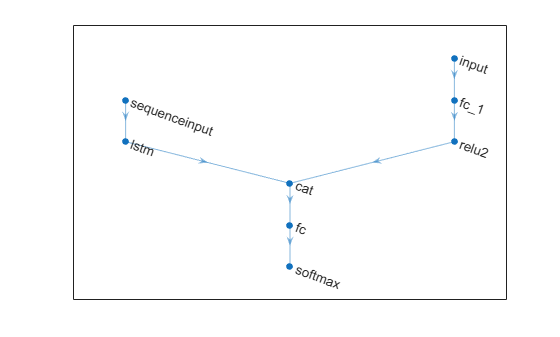You can replace the LSTM layer with a block of layers that processes vector sequence data. This layer maps `"CBT"` (channel, batch, time) data to `"CB"` (channel, batch) data.

You can replace the fully connected layer and ReLU layer in the feature branch with a block of layers that processes feature data. This block maps `"CB"` (channel, batch) data to `"CB"` (channel, batch) data.